# How to fill NAN values with mean in Pandas?

• Difficulty Level : Easy
• Last Updated : 24 Jan, 2021

It is a quite compulsory process to modify the data we have as the computer will show you an error of invalid input as it is quite impossible to process the data having ‘NaN’ with it and it is not quite practically possible to manually change the ‘NaN’ to its mean. Therefore, to resolve this problem we process the data and use various functions by which the ‘NaN’ is removed from our data and is replaced with the particular mean and ready be get process by the system.

Mainly there are two steps to remove ‘NaN’ from the data-

1. Using  Dataframe.fillna()  from the pandas’ library.
2. Using  SimpleImputer from sklearn.impute (this is only useful if the data is present in the form of csv file)

### Using  Dataframe.fillna()  from the pandas’ library

With the help of Dataframe.fillna()  from the pandas’ library, we can easily replace the ‘NaN’ in the data frame.

Procedure:

1. To calculate the mean() we use the mean function of the particular column
2. Now with the help of fillna() function we will change all ‘NaN’ of that particular column for which we have its mean.
3. We will print the updated column.

Syntax: df.fillna(value=None, method=None, axis=None, inplace=False, limit=None, downcast=None, **kwargs)

Parameter:

• value : Value to use to fill holes
• method : Method to use for filling holes in reindexed Series pad / fill
• axis : {0 or ‘index’}
• inplace : If True, fill in place.
• limit : If method is specified, this is the maximum number of consecutive NaN values to forward/backward fill
• downcast : dict, default is None

Example 1:

1. To calculate the mean() we use the mean function of the particular column
2. Then apply fillna() function, we will change all ‘NaN’ of that particular column for which we have its mean and print the updated data frame.

## Python3

 `import` `numpy as np``import` `pandas as pd`` ` `# A dictionary with list as values``GFG_dict ``=` `{ ``'G1'``: [``10``, ``20``,``30``,``40``],``                ``'G2'``: [``25``, np.NaN, np.NaN, ``29``],``                ``'G3'``: [``15``, ``14``, ``17``, ``11``],``                ``'G4'``: [``21``, ``22``, ``23``, ``25``]}`` ` `# Create a DataFrame from dictionary``gfg ``=` `pd.DataFrame(GFG_dict)`` ` `#Finding the mean of the column having NaN``mean_value``=``gfg[``'G2'``].mean()`` ` `# Replace NaNs in column S2 with the``# mean of values in the same column``gfg[``'G2'``].fillna(value``=``mean_value, inplace``=``True``)``print``(``'Updated Dataframe:'``)``print``(gfg)`

Output: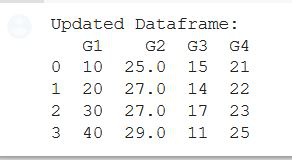Example 2:

## Python3

 `import` `pandas as pd``import` `numpy as np`` ` `df ``=` `pd.DataFrame({``    ``'ID'``: [``10``, np.nan, ``20``, ``30``, np.nan, ``50``, np.nan,``           ``150``, ``200``, ``102``, np.nan, ``130``],``     ` `    ``'Sale'``: [``10``, ``20``, np.nan, ``11``, ``90``, np.nan,``             ``55``, ``14``, np.nan, ``25``, ``75``, ``35``],``     ` `    ``'Date'``: [``'2020-10-05'``, ``'2020-09-10'``, np.nan,``             ``'2020-08-17'``, ``'2020-09-10'``, ``'2020-07-27'``, ``             ``'2020-09-10'``, ``'2020-10-10'``, ``'2020-10-10'``,``             ``'2020-06-27'``, ``'2020-08-17'``, ``'2020-04-25'``],``})`` ` `df[``'Sale'``].fillna(``int``(df[``'Sale'``].mean()), inplace``=``True``)``print``(df)`

Output: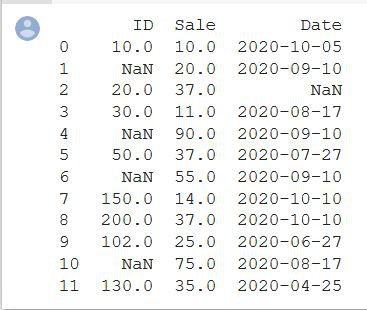### Using  SimpleImputer() from sklearn.impute

This function Imputation transformer for completing missing values which provide basic strategies for imputing missing values. These values can be imputed with a provided constant value or using the statistics (mean, median, or most frequent) of each column in which the missing values are located. This class also allows for different missing value encoding.

Syntax: class sklearn.impute.SimpleImputer(*, missing_values=nan, strategy=’mean’, fill_value=None, verbose=0, copy=True, add_indicator=False)

Parameters:

• missing_values: int float, str, np.nan or None, default=np.nan
• strategy string: default=’mean’
• fill_valuestring or numerical value: default=None
• verbose: integer, default=0
• copy: boolean, default=True
• add_indicator: boolean, default=False

Note : Data Used in below examples is here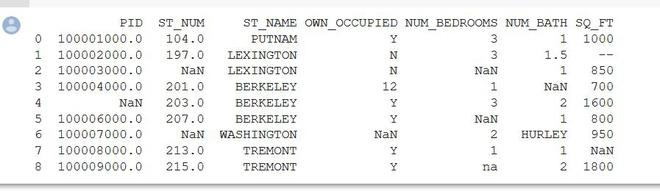Example 1 : (Computation on PID column)

## Python3

 `import` `pandas as pd``import` `numpy as np`` ` `Dataset``=` `pd.read_csv(``"property data.csv"``)``X ``=` `Dataset.iloc[:,``0``].values`` ` `# To calculate mean use imputer class``from` `sklearn.impute ``import` `SimpleImputer``imputer ``=` `SimpleImputer(missing_values``=``np.nan, strategy``=``'mean'``)``imputer ``=` `imputer.fit(X)`` ` `X ``=` `imputer.transform(X)``print``(X)`

Output: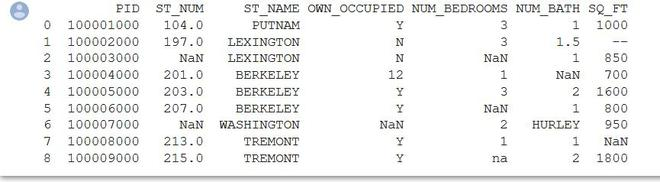Example 2 : (Computation on ST_NUM column)

## Python3

 `from` `sklearn.impute ``import` `SimpleImputer``import` `pandas as pd``import` `numpy as np`` ` `Dataset ``=` `pd.read_csv(``"property data.csv"``)``X ``=` `Dataset.iloc[:, ``1``].values`` ` `# To calculate mean use imputer class``imputer ``=` `SimpleImputer(missing_values``=``np.nan, strategy``=``'mean'``)``imputer ``=` `imputer.fit(X)``X ``=` `imputer.transform(X)``print``(X)`

Output: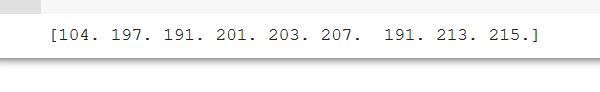My Personal Notes arrow_drop_up